# Tension Calculator

0m

1,000m

meters
feet

0m

150m

meters
feet

0kg

150kg

kg
lbs

## Tension

Approximation Exact

## The Math

Before we begin, it's worth noting that the value calculated with these formulas is independent of the webbing type. The reason for this is because we are calculating the value of the force when a person or weight is applied to the line in the middle. At this moment in time, there is theoretically no movement in the system. Line dynamics do not come into play in this scenario. When there is no one on the line, the force in the system greatly depends on the material type and how much it elongates at a given force (stress-strain curve). We are not calculating this value though.

To begin, let's take a look at a diagram of what a slackline looks like when someone is standing in the middle.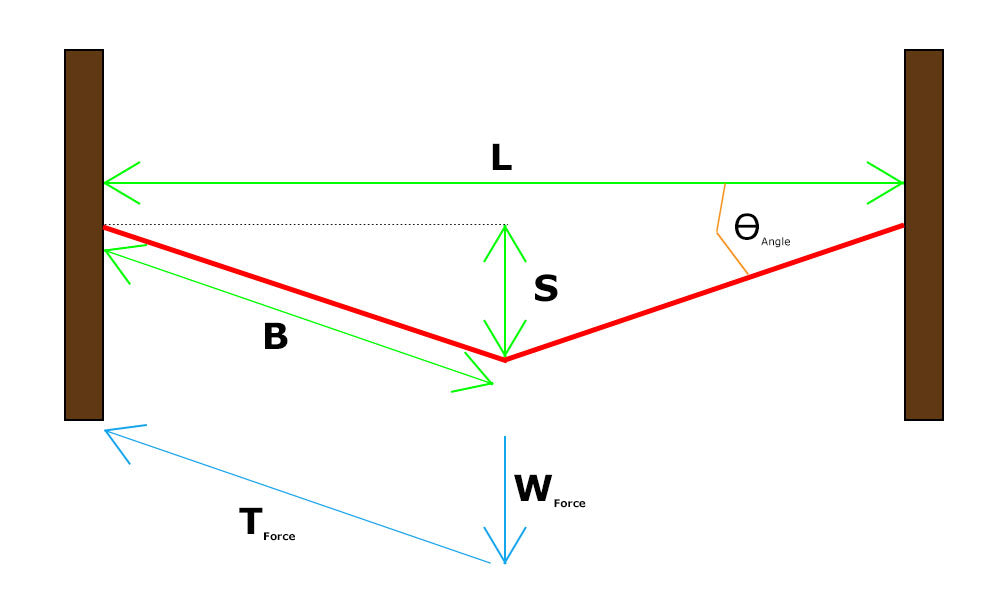• L = Line Length
• S = Line Sag
• B = Half of line length after standing on the line
• Θ = Angle between original line and line after standing on it
• TForce = Tension in the line
• WForce = Weight of the slackliner

From the above, we are trying to find the value of TForce. Now, keep in mind, your body weight is shared amongst the two anchors. With that information, we can deconstruction the above diagram into a simple triangle, which we can compute the tension easily.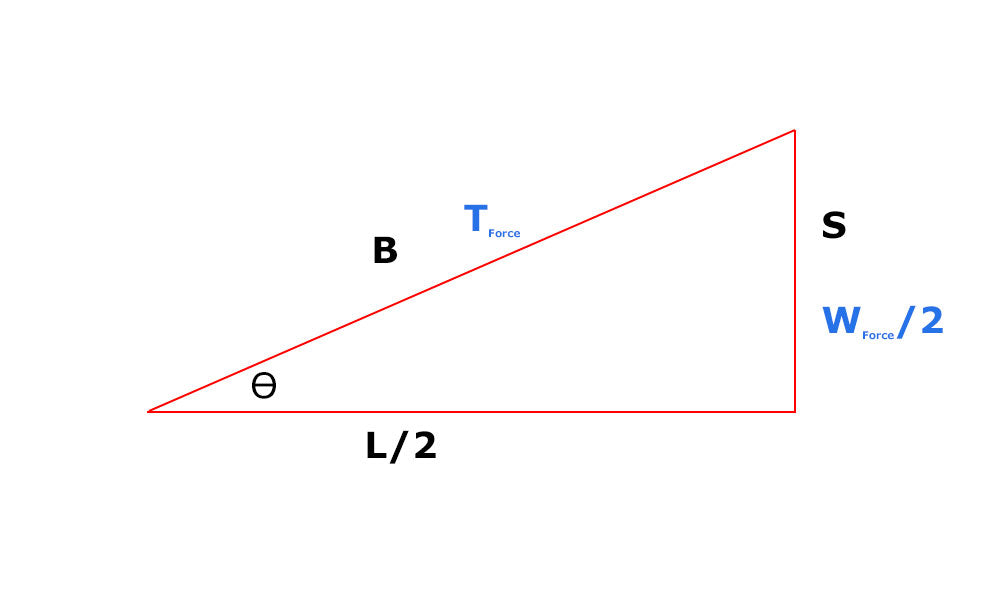From this diagram we can easily see that the following is true:We can also derive the following via the Pythagorean Theorem: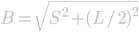Now using the old "Two Equations, Two Unknowns" trick, we can easily solve for T.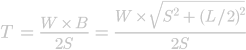That will give us the exact value for the tension. However, there is a MUCH easier way to calculate this value! If you notice, the Θvalue is extremely small due to the large difference between the length and sag. For this reason, we can see that the value of Bis extremely close to the value of L/2. So, what if we substitute one for the other in the above equations?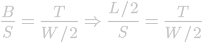That leaves us with only 1 unknown...T! Let's solve for it :)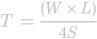That will give us the approximate force on the slackline. This approximation will work for most scenarios where the length of the line is much greater than the sag. When you start getting into rodeolines or super loose lines (sag-to-length ratio more than 1/5), you will start to see a bigger difference between the approximate and actual values.

You've just added this product to the cart:#price#
×